### Home > INT2 > Chapter 12 > Lesson 12.1.3 > Problem12-34

12-34.
1. What do you think 0! is equal to? Homework Help ✎

1. Try it on your calculator to see what you get.

2. What does 8P8 mean? What should 8P8 be equal to? Write 8P8 using the factorial formula. Why is it necessary for 0! to equal 1?

3. Do you remember how to show that 20 = 1? You can use a sequence of powers of two like this:= 23,= 22,= 21, so= 20. Since= 1 you also know that 20 = 1.

You can construct a similar pattern for 0!, starting with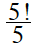= 4! and then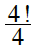= 3!. Continue the pattern and make an argument to justify that 0! = 1.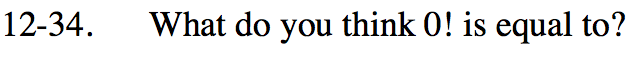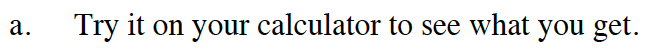1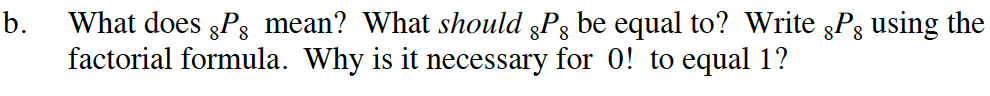Refer to the Math Notes box for help.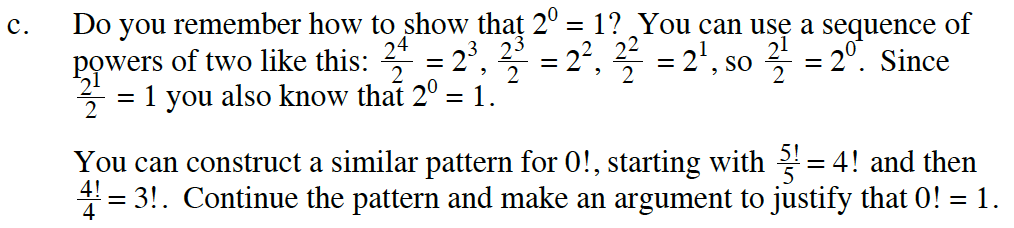$\frac{3!}{3} - 2!, \frac{2!}{2} = 1, \frac{1!}{1} = 0!$

$\frac{1!}{1} = \frac{1}{1} = 1 = 0!$